# A closer look at orbit integration¶

## Orbit initialization¶

### Standard initialization¶

Orbits can be initialized in various coordinate frames. The simplest initialization gives the initial conditions directly in the Galactocentric cylindrical coordinate frame (or in the rectangular coordinate frame in one dimension). Orbit() automatically figures out the dimensionality of the space from the initial conditions in this case. In three dimensions initial conditions are given either as vxvv=[R,vR,vT,z,vz,phi] or one can choose not to specify the azimuth of the orbit and initialize with vxvv=[R,vR,vT,z,vz]. Since potentials in galpy are easily initialized to have a circular velocity of one at a radius equal to one, initial coordinates are best given as a fraction of the radius at which one specifies the circular velocity, and initial velocities are best expressed as fractions of this circular velocity. For example,

>>> o= Orbit(vxvv=[1.,0.1,1.1,0.,0.1,0.])


initializes a fully three-dimensional orbit, while

>>> o= Orbit(vxvv=[1.,0.1,1.1,0.,0.1])


initializes an orbit in which the azimuth is not tracked, as might be useful for axisymmetric potentials.

In two dimensions, we can similarly specify fully two-dimensional orbits o=Orbit(vxvv=[R,vR,vT,phi]) or choose not to track the azimuth and initialize with o= Orbit(vxvv=[R,vR,vT]).

In one dimension we simply initialize with o= Orbit(vxvv=[x,vx]).

### Initialization with physical scales¶

Orbits are normally used in galpy’s natural coordinates. When Orbits are initialized using a distance scale ro= and a velocity scale vo=, then many Orbit methods return quantities in physical coordinates. Specifically, physical distance and velocity scales are specified as

>>> op= Orbit(vxvv=[1.,0.1,1.1,0.,0.1,0.],ro=8.,vo=220.)


All output quantities will then be automatically be specified in physical units: kpc for positions, km/s for velocities, (km/s)^2 for energies and the Jacobi integral, km/s kpc for the angular momentum o.L() and actions, 1/Gyr for frequencies, and Gyr for times and periods. See below for examples of this.

Physical units are only used for outputs: internally natural units are still used and inputs have to also be specified in natural units (for example, integration times or the time at which an output is requested must be specified in natural units). If for any output you do not want the output in physical units, you can specify this by supplying the keyword argument use_physical=False.

### Initialization from observed coordinates¶

For orbit integration and characterization of observed stars or clusters, initial conditions can also be specified directly as observed quantities when radec=True is set. In this case a full three-dimensional orbit is initialized as o= Orbit(vxvv=[RA,Dec,distance,pmRA,pmDec,Vlos],radec=True) where RA and Dec are expressed in degrees, the distance is expressed in kpc, proper motions are expressed in mas/yr (pmra = pmra’ * cos[Dec] ), and Vlos is the heliocentric line-of-sight velocity given in km/s. The observed epoch is currently assumed to be J2000.00. These observed coordinates are translated to the Galactocentric cylindrical coordinate frame by assuming a Solar motion that can be specified as either solarmotion=hogg (default; 2005ApJ...629..268H), solarmotion=dehnen (1998MNRAS.298..387D) or solarmotion=shoenrich (2010MNRAS.403.1829S). A circular velocity can be specified as vo=220 in km/s and a value for the distance between the Galactic center and the Sun can be given as ro=8.0 in kpc (e.g., 2012ApJ...759..131B). While the inputs are given in physical units, the orbit is initialized assuming a circular velocity of one at the distance of the Sun (that is, the orbit’s position and velocity is scaled to galpy’s natural units after converting to the Galactocentric coordinate frame, using the specified ro= and vo=). The parameters of the coordinate transformations are stored internally, such that they are automatically used for relevant outputs (for example, when the RA of an orbit is requested). An example of all of this is:

>>> o= Orbit(vxvv=[20.,30.,2.,-10.,20.,50.],radec=True,ro=8.,vo=220.)


However, the internally stored position/velocity vector is

>>> print o.vxvv
[1.1476649101960512, 0.20128601278731811, 1.8303776114906387, -0.13107066602923434, 0.58171049004255293, 0.14071341020496472]


and is therefore in natural units.

Similarly, one can also initialize orbits from Galactic coordinates using o= Orbit(vxvv=[glon,glat,distance,pmll,pmbb,Vlos],lb=True), where glon and glat are Galactic longitude and latitude expressed in degrees, and the proper motions are again given in mas/yr ((pmll = pmll’ * cos[glat] ):

>>> o= Orbit(vxvv=[20.,30.,2.,-10.,20.,50.],lb=True,ro=8.,vo=220.)
>>> print o.vxvv
[0.79998509943955398, 0.075939950035477488, 0.52838231795389867, 0.12812499999999999, 0.89052135379600328, 0.092696334097541536]


When radec=True or lb=True is set, velocities can also be specified in Galactic coordinates if UVW=True is set. The input is then vxvv=[RA,Dec,distance,U,V,W], where the velocities are expressed in km/s. U is, as usual, defined as -vR (minus vR).

When orbits are initialized using radec=True or lb=True, physical scales ro= and vo= are automatically specified (because they have defaults of ro=8 and vo=220). Therefore, all output quantities will be specified in physical units (see above). If you do want to get outputs in galpy’s natural coordinates, you can turn this behavior off by doing

>>> o.turn_physical_off()


All outputs will then be specified in galpy’s natural coordinates.

## Orbit integration¶

After an orbit is initialized, we can integrate it for a set of times ts, given as a numpy array. For example, in a simple logarithmic potential we can do the following

>>> from galpy.potential import LogarithmicHaloPotential
>>> lp= LogarithmicHaloPotential(normalize=1.)
>>> o= Orbit(vxvv=[1.,0.1,1.1,0.,0.1,0.])
>>> import numpy
>>> ts= numpy.linspace(0,100,10000)
>>> o.integrate(ts,lp)


to integrate the orbit from t=0 to t=100, saving the orbit at 10000 instances.

If we initialize the Orbit using a distance scale ro= and a velocity scale vo=, then Orbit plots and outputs will use physical coordinates (currently, times, positions, and velocities)

>>> op= Orbit(vxvv=[1.,0.1,1.1,0.,0.1,0.],ro=8.,vo=220.) #Use Vc=220 km/s at R= 8 kpc as the normalization
>>> op.integrate(ts,lp) #times are still specified in natural coordinates


## Displaying the orbit¶

After integrating the orbit, it can be displayed by using the plot() function. The quantities that are plotted when plot() is called depend on the dimensionality of the orbit: in 3D the (R,z) projection of the orbit is shown; in 2D either (X,Y) is plotted if the azimuth is tracked and (R,vR) is shown otherwise; in 1D (x,vx) is shown. E.g., for the example given above,

>>> o.plot()


gives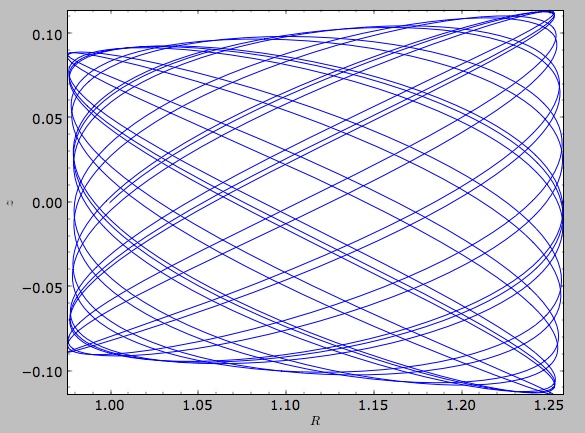If we do the same for the Orbit that has physical distance and velocity scales associated with it, we get the following

>>> op.plot()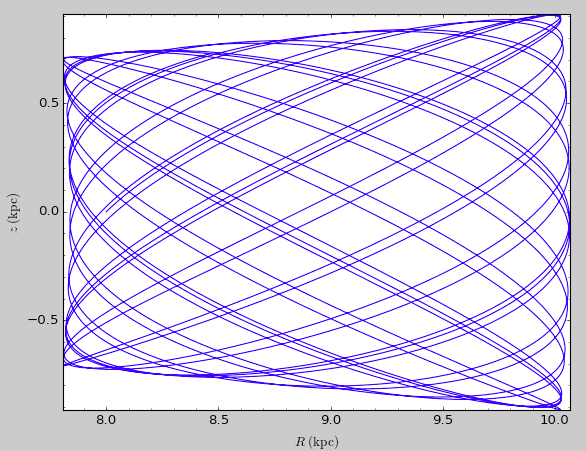If we call op.plot(use_physical=False), the quantities will be displayed in natural galpy coordinates.

Other projections of the orbit can be displayed by specifying the quantities to plot. E.g.,

>>> o.plot(d1='x',d2='y')


gives the projection onto the plane of the orbit: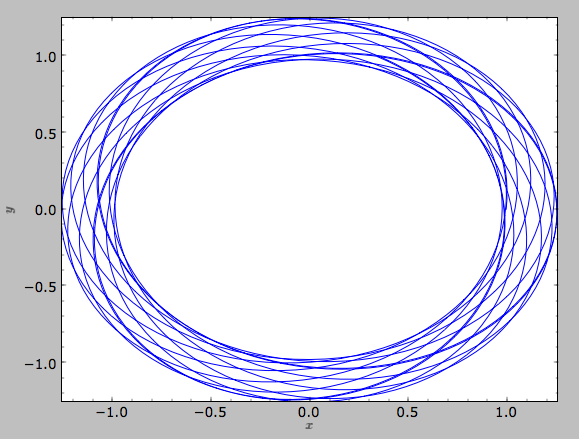while

>>> o.plot(d1='R',d2='vR')


gives the projection onto (R,vR):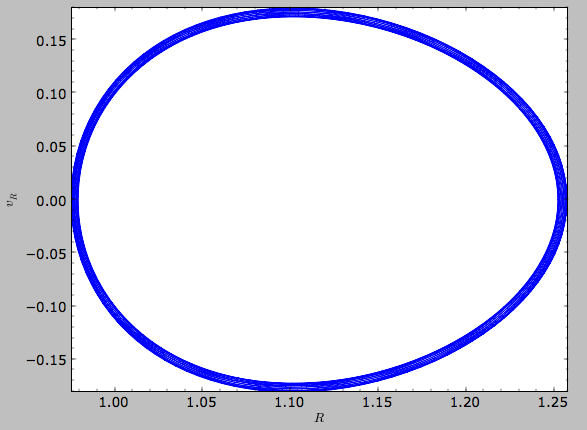We can also plot the orbit in other coordinate systems such as Galactic longitude and latitude

>>> o.plot('k.',d1='ll',d2='bb')


which shows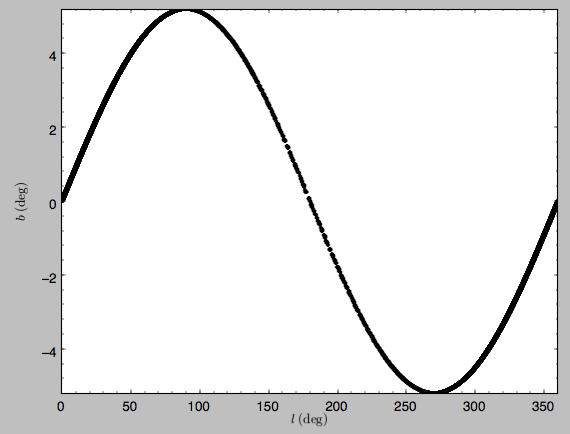or RA and Dec

>>> o.plot('k.',d1='ra',d2='dec')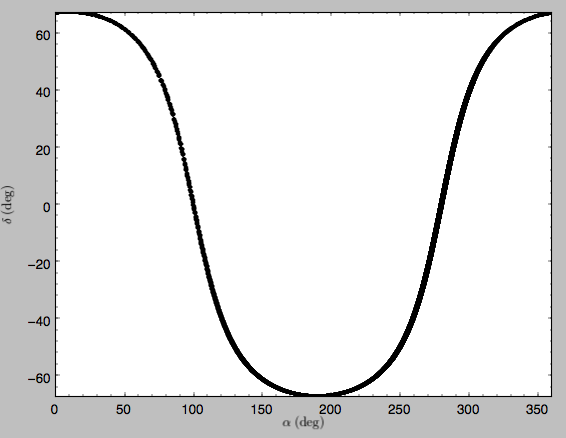See the documentation of the o.plot function and the o.ra(), o.ll(), etc. functions on how to provide the necessary parameters for the coordinate transformations.

## Orbit characterization¶

The properties of the orbit can also be found using galpy. For example, we can calculate the peri- and apocenter radii of an orbit, its eccentricity, and the maximal height above the plane of the orbit

>>> o.rap(), o.rperi(), o.e(), o.zmax()
(1.2581455175173673,0.97981663263371377,0.12436710999105324,0.11388132751079502)


We can also calculate the energy of the orbit, either in the potential that the orbit was integrated in, or in another potential:

>>> o.E(), o.E(pot=mp)
(0.6150000000000001, -0.67390625000000015)


where mp is the Miyamoto-Nagai potential of Introduction: Rotation curves.

For the Orbit op that was initialized above with a distance scale ro= and a velocity scale vo=, these outputs are all in physical units

>>> op.rap(), op.rperi(), op.e(), op.zmax()
(10.065158988860341,7.8385312810643057,0.12436696983841462,0.91105035688072711) #kpc
>>> op.E(), op.E(pot=mp)
(29766.000000000004, -32617.062500000007) #(km/s)^2


We can also show the energy as a function of time (to check energy conservation)

>>> o.plotE(normed=True)


gives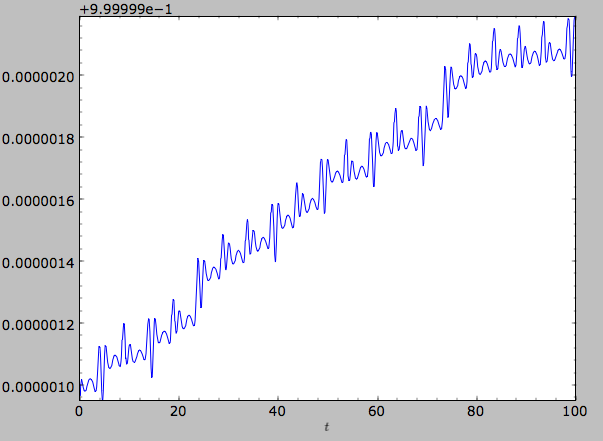We can specify another quantity to plot the energy against by specifying d1=. We can also show the vertical energy, for example, as a function of R

>>> o.plotEz(d1='R',normed=True)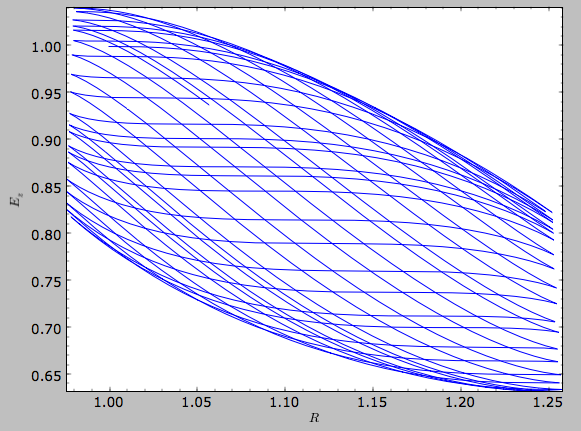Often, a better approximation to an integral of the motion is given by Ez/sqrt(density[R]). We refer to this quantity as EzJz and we can plot its behavior

>>> o.plotEzJz(d1='R',normed=True)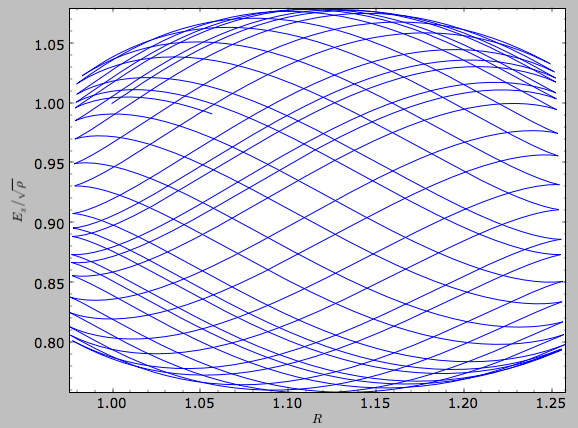## Accessing the raw orbit¶

The value of R, vR, vT, z, vz, x, vx, y, vy, phi, and vphi at any time can be obtained by calling the corresponding function with as argument the time (the same holds for other coordinates ra, dec, pmra, pmdec, vra, vdec, ll, bb, pmll, pmbb, vll, vbb, vlos, dist, helioX, helioY, helioZ, U, V, and W). If no time is given the initial condition is returned, and if a time is requested at which the orbit was not saved spline interpolation is used to return the value. Examples include

>>> o.R(1.)
1.1545076874679474
>>> o.phi(99.)
88.105603035901169
>>> o.ra(2.,obs=[8.,0.,0.],ro=8.)
array([ 285.76403985])
>>> o.helioX(5.)
array([ 1.24888927])
>>> o.pmll(10.,obs=[8.,0.,0.,0.,245.,0.],ro=8.,vo=230.)
array([-6.45263888])


For the Orbit op that was initialized above with a distance scale ro= and a velocity scale vo=, the first of these would be

>>> op.R(1.)
9.2360614837829225 #kpc


which we can also access in natural coordinates as

>>> op.R(1.,use_physical=False)
1.1545076854728653


We can also specify a different distance or velocity scale on the fly, e.g.,

>>> op.R(1.,ro=4.) #different velocity scale would be vo=
4.6180307418914612


We can also initialize an Orbit instance using the phase-space position of another Orbit instance evaulated at time t. For example,

>>> newOrbit= o(10.)


will initialize a new Orbit instance with as initial condition the phase-space position of orbit o at time=10..

The whole orbit can also be obtained using the function getOrbit

>>> o.getOrbit()


which returns a matrix of phase-space points with dimensions [ntimes,ndim].

## Fast orbit integration¶

The standard orbit integration is done purely in python using standard scipy integrators. When fast orbit integration is needed for batch integration of a large number of orbits, a set of orbit integration routines are written in C that can be accessed for most potentials, as long as they have C implementations, which can be checked by using the attribute hasC

>>> mp= MiyamotoNagaiPotential(a=0.5,b=0.0375,amp=1.,normalize=1.)
>>> mp.hasC
True


Fast C integrators can be accessed through the method= keyword of the orbit.integrate method. Currently available integrators are

• rk4_c
• rk6_c
• dopr54_c

which are Runge-Kutta and Dormand-Prince methods. There are also a number of symplectic integrators available

• leapfrog_c
• symplec4_c
• symplec6_c

The higher order symplectic integrators are described in Yoshida (1993).

For most applications I recommend dopr54_c. For example, compare

>>> o= Orbit(vxvv=[1.,0.1,1.1,0.,0.1])
>>> timeit(o.integrate(ts,mp))
1 loops, best of 3: 553 ms per loop
>>> timeit(o.integrate(ts,mp,method='dopr54_c'))
galpyWarning: Using C implementation to integrate orbits
10 loops, best of 3: 25.6 ms per loop


As this example shows, galpy will issue a warning that C is being used. Speed-ups by a factor of 20 are typical.

## Integration of the phase-space volume¶

galpy further supports the integration of the phase-space volume through the method integrate_dxdv, although this is currently only implemented for two-dimensional orbits (planarOrbit). As an example, we can check Liouville’s theorem explicitly. We initialize the orbit

>>> o= Orbit(vxvv=[1.,0.1,1.1,0.])


and then integrate small deviations in each of the four phase-space directions

>>> ts= numpy.linspace(0.,28.,1001) #~1 Gyr at the Solar circle
>>> o.integrate_dxdv([1.,0.,0.,0.],ts,mp,method='dopr54_c',rectIn=True,rectOut=True)
>>> dx= o.getOrbit_dxdv()[-1,:] # evolution of dxdv along the orbit
>>> o.integrate_dxdv([0.,1.,0.,0.],ts,mp,method='dopr54_c',rectIn=True,rectOut=True)
>>> dy= o.getOrbit_dxdv()[-1,:]
>>> o.integrate_dxdv([0.,0.,1.,0.],ts,mp,method='dopr54_c',rectIn=True,rectOut=True)
>>> dvx= o.getOrbit_dxdv()[-1,:]
>>> o.integrate_dxdv([0.,0.,0.,1.],ts,mp,method='dopr54_c',rectIn=True,rectOut=True)
>>> dvy= o.getOrbit_dxdv()[-1,:]


We can then compute the determinant of the Jacobian of the mapping defined by the orbit integration from time zero to the final time

>>> tjac= numpy.linalg.det(numpy.array([dx,dy,dvx,dvy]))


This determinant should be equal to one

>>> print tjac
0.999999991189
>>> numpy.fabs(tjac-1.) < 10.**-8.
True


The calls to integrate_dxdv above set the keywords rectIn= and rectOut= to True, as the default input and output uses phase-space volumes defined as (dR,dvR,dvT,dphi) in cylindrical coordinates. When rectIn or rectOut is set, the in- or output is in rectangular coordinates ([x,y,vx,vy] in two dimensions).

Implementing the phase-space integration for three-dimensional FullOrbit instances is straightforward and is part of the longer term development plan for galpy. Let the main developer know if you would like this functionality, or better yet, implement it yourself in a fork of the code and send a pull request!

## Example: The eccentricity distribution of the Milky Way’s thick disk¶

A straightforward application of galpy’s orbit initialization and integration capabilities is to derive the eccentricity distribution of a set of thick disk stars. We start by downloading the sample of SDSS SEGUE (2009AJ....137.4377Y) thick disk stars compiled by Dierickx et al. (2010arXiv1009.1616D) at

http://www.mpia-hd.mpg.de/homes/rix/Data/Dierickx-etal-tab2.txt

After reading in the data (RA,Dec,distance,pmRA,pmDec,vlos; see above) as a vector vxvv with dimensions [6,ndata] we (a) define the potential in which we want to integrate the orbits, and (b) integrate each orbit and save its eccentricity (running this for all 30,000-ish stars will take about half an hour)

>>> lp= LogarithmicHaloPotential(normalize=1.)
>>> ts= nu.linspace(0.,20.,10000)
>>> mye= nu.zeros(ndata)
>>> for ii in range(len(e)):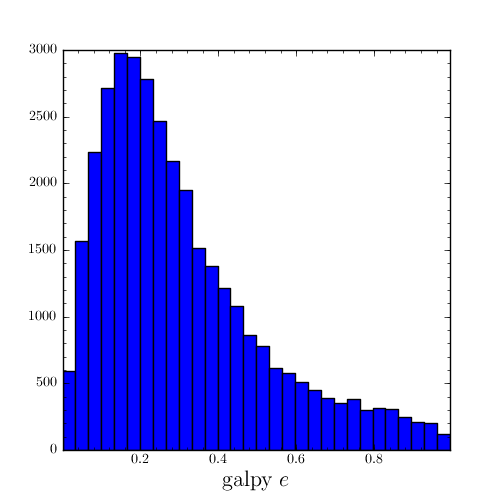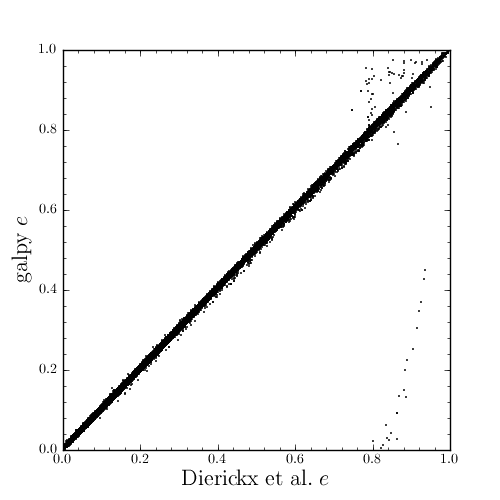The script that calculates and plots everything can be downloaded here.